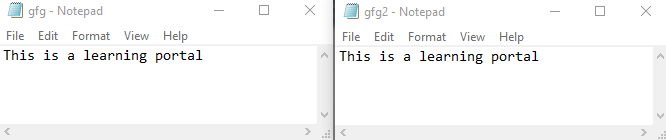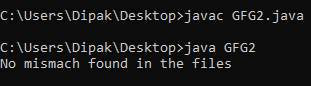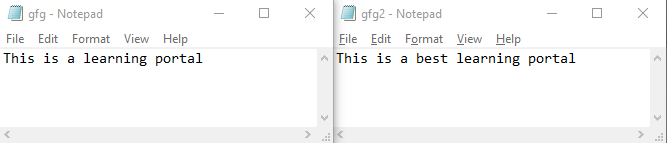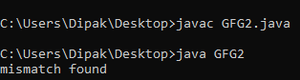# mismatch() Method of java.nio.file.Files Utility Class with Examples

mismatch() method is newly added in java jdk-12 in the java.nio.file.Files class. This method is mainly used to compare each byte of data of two files from start and return the point at which the bit differs. If the bit doesn’t differ at any point then it returns “-1L” indicating that files are the same and exact copies of each other.

This method mainly takes the paths of the files as its input and returns a long value indicating the position of bit mismatch. So the declaration of this method is as shown:

`long position = Files.mismatch(Path path1, Path path2);`

This method requires that the two files two be compared should have the correct path given and their sizes should be the same along with the format.

Example 1:

Files were:## Java

 `// Java program to demonstrate the usage` `// of mismatch() method`   `import` `java.io.IOException;` `import` `java.nio.file.Files;` `import` `java.nio.file.Path;` `import` `java.util.*;`   `// save file as the name of GFG2` `public` `class` `GFG2 {` `  `  `    ``// main class` `    ``public` `static` `void` `main(String[] args) ``throws` `Exception` `    ``{` `        ``// getting the file path to the respective two files` `        ``Path filePath1 = Path.of(``"C:\\Users\\Dipak\\Desktop\\gfg.txt"``);` `        ``Path filePath2 = Path.of(``"c:\\Users\\Dipak\\Desktop\\gfg2.txt"``);` `      `  `        ``// calling the mismatchfunction` `        ``long` `mis_match = Files.mismatch(filePath1, filePath2);` `      `  `        ``// printing the output result.` `        ``if` `(mis_match == -``1``)` `            ``System.out.println(``"No mismatch found in the files"``);` `        ``else` `            ``System.out.println(``"mismatch found"``);` `    ``}` `}`

Output:Example 2:

Files were:## Java

 `// Java program to demonstrate the` `// usage of mismatch() method`   `import` `java.io.IOException;` `import` `java.nio.file.Files;` `import` `java.nio.file.Path;` `import` `java.util.*;`   `// save file as the name of GFG2` `public` `class` `GFG2 {`   `    ``// main class` `    ``public` `static` `void` `main(String[] args) ``throws` `Exception` `    ``{` `        ``// getting the file path to the respective two files` `        ``Path filePath1 = Path.of(``"C:\\Users\\Dipak\\Desktop\\gfg.txt"``);` `        ``Path filePath2 = Path.of(``"c:\\Users\\Dipak\\Desktop\\gfg2.txt"``);` `      `  `        ``// calling the mismatchfunction` `        ``long` `mis_match = Files.mismatch(filePath1, filePath2);` `      `  `        ``// printing the output result.` `        ``if` `(mis_match == -``1``)` `            ``System.out.println(``"No mismatch found in the files"``);` `        ``else` `            ``System.out.println(``"mismatch found"``);` `    ``}` `}`

Output:output